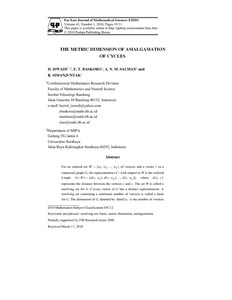# The Metric Dimension of Amalgamation of Cycles

Iswadi, Hazrul and Baskoro, Edy Tri and Salman, A.N.M and Simanjuntak, Rinovia (2010) The Metric Dimension of Amalgamation of Cycles. Far East Journal of Mathematical Sciences (FJMS), 41 (1). pp. 19-31. ISSN 0972-0871Preview PDF hazrul_The Metric Dimension of Amalgamation of Cycles_2010.pdf Download (298kB) | Preview
Official URL / DOI: http://pphmj.com/journals/fjms.htm

## Abstract

For an ordered set W = {w_1, w_2 , ..., w_k } of vertices and a vertex v in a connected graph G, the representation of v with respect to W is the ordered k-tuple r(v|W) = (d(v,w_1), d(v,w_2 ), ..., d (v,w_k )), where d(x,y) represents the distance between the vertices x and y. The set W is called a resolving set for G if every vertex of G has a distinct representation. A resolving set containing a minimum number of vertices is called a basis for G. The dimension of G, denoted by dim(G), is the number of vertices in a basis of G. Let {G_i} be a finite collection of graphs and each G_i has a fixed vertex voi called a terminal. The amalgamation Amal {Gi , v_{oi}} is formed by taking all of the G_i’s and identifying their terminals. In this paper, we determine the metric dimension of amalgamation of cycles.

Item Type: Article Amalgamation, Cycles, Resolving set, Metric Dimension Q Science > QA Mathematics Academic Department > Department of Mathematics and Natural Science Hazrul Iswadi 6179 08 Mar 2012 07:03 20 Mar 2012 01:43 http://repository.ubaya.ac.id/id/eprint/172View Item